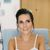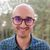# QlikView App Dev

Discussion Board for collaboration related to QlikView App Development.

Announcements
Join “Do More With Qlik” with Mike Tarallo to get a first-hand look at the New Authoring Experience, June 29th: REGISTER HERE
cancel
Showing results for
Did you mean:Creator III

## set a variable

hi,
i want to set a variable in the script that have-
if(\$(v_metric_5)<90,'red',if(\$(v_metric_5)<95,'yellow',if(\$(v_metric_5)<=100,'green','gray')))

how can i do that?

i dont want that \$(v_metric_5) will calculate

i want it to stay as a string

4 RepliesMVP

Not sure, what do you mean by string? May be this

if('\$(v_metric_5)'<90,'red',if('\$(v_metric_5)'<95,'yellow',if('\$(v_metric_5)'<=100,'green','gray')))

How you have created this variable v_metric_5

Before develop something, think If placed (The Right information | To the right people | At the Right time | In the Right place | With the Right context)Creator III

yes what is that v_metric_5 ??

is it is a variable ?? if varible then it will take the values as per the variable value.

if it is field  then you no need to consider it as string or any other format...Partner

Here is the difference between setting the variables:

SET vVariable = 1 + 2; //Value returned 1 + 2

LET vVariable = 1 + 2; //Value returned 3

See the attachment that might be helpful.MVP

Dollar Sign Expansion (DSE) always occurs in script, which is generally a blessing but sometimes a curse.  One workaround is to use a proxy character for the \$ and then replace(). For example:

LET v1 = replace(

'if(@(v_metric_5)<90,''red'',if(@(v_metric_5)<95,''yellow'',if(@(v_metric_5)<=100,''green'',''gray'')))'

,'@','\$');

You will need to double up your single quotes to allow them within the LET string.

Another approach is to use a proxy char for both \$ and ' replacing both with a mapsubstring:

EscapeCharsMap:

from, to

@,\$

|,'

];

LET v2 = MapSubString('EscapeCharsMap',

'if(@(v_metric_5)<90,|red|,if(@(v_metric_5)<95,|yellow|,if(@(v_metric_5)<=100,|green|,|gray|)))'

);

-Rob